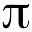[Ben]:Handy Excel Macro - Random Selection of X Number of Records Discuss This [3 comments so far] View Comments
One of my coworkers needed a macro written to speed up random selection from within a file. This is what I came up with. This is tested with Excel 2007 and will need tweaking to work with earlier versions. It works with any number of columns, asks you how many rows you want and asks if you have a header row.

 Sub a_RandomSelect()     Application.ScreenUpdating = False 'disable screen updating to avoid screen flashing     Application.DisplayAlerts = False          Dim wbk1 As Workbook, wbk2 As Workbook, lstRow As Long, wks As Worksheet, startCut As Integer, selNum As Integer, lstCol As String          Set wbk1 = ActiveWorkbook     Set wks = wbk1.ActiveSheet     maxRng = "953360"     If wks.Range("A1").CurrentRegion.Rows.Count > 0 Then         If wks.Range("A1").CurrentRegion.Rows.Count - 1 > 0 Then             lstRow = wks.Range("A" & maxRng).End(xlUp).Row             ' get number of rows             selNum = InputBox("How many records do you want to select?")             ' determine if a header row exists             hdrRsp = MsgBox("Do you have a header row?", xlQuestion + vbYesNo, "Header Row Question")             If hdrRsp = vbYes Then                 hdrYesNo = xlYes             Else                 hdrYesNo = xlNo             End If             ' add Random column             wks.Columns("A").Insert Shift:=xlToRight             wks.Range("A1").Formula = "=RAND()"             wks.Range("A1").AutoFill Destination:=wks.Range("A1:A" & lstRow)             ' copy with values only             wks.Range("A:A").Copy             wks.Range("A:A").PasteSpecial (xlPasteValues)             ' sort by random             lstCol = Cells.Find(What:="*", After:=[A1], SearchDirection:=xlPrevious).Column             With ActiveWorkbook.Worksheets(ActiveSheet.Name).Sort                 .SortFields.Clear                 .SortFields.Add Key:=Range("A:A"), _                 SortOn:=xlSortOnValues, Order:=xlAscending, DataOption:=xlSortNormal                 .SetRange Range("A1:" & GetColumnLetter(CInt(lstCol)) & lstRow)                 .Header = hdrYesNo                 .MatchCase = False                 .Orientation = xlTopToBottom                 .SortMethod = xlPinYin                 .Apply             End With             ' Delete any records beyond selNum+header+1             If hdrYesNo = xlYes Then                 startCut = selNum + 2             Else                 startCut = selNum + 1             End If             wks.Range("A" & startCut & ":" & GetColumnLetter(CInt(lstCol)) & lstRow).Delete             ' Remove First Column             wks.Range("A:A").Delete             wks.Range("A1").Select         End If     End If     Application.DisplayAlerts = True     Application.ScreenUpdating = True     MsgBox selNum & " Selected" End Sub
2010-01-22
Handy Excel Macro - Random Selection of X Number of Records

BenSwenson.com Home
ForumsRegister here
 ©2023 by Ben Swenson.   All rights reserved.Reproduction in whole or in part without permission is prohibited. Hosted by: